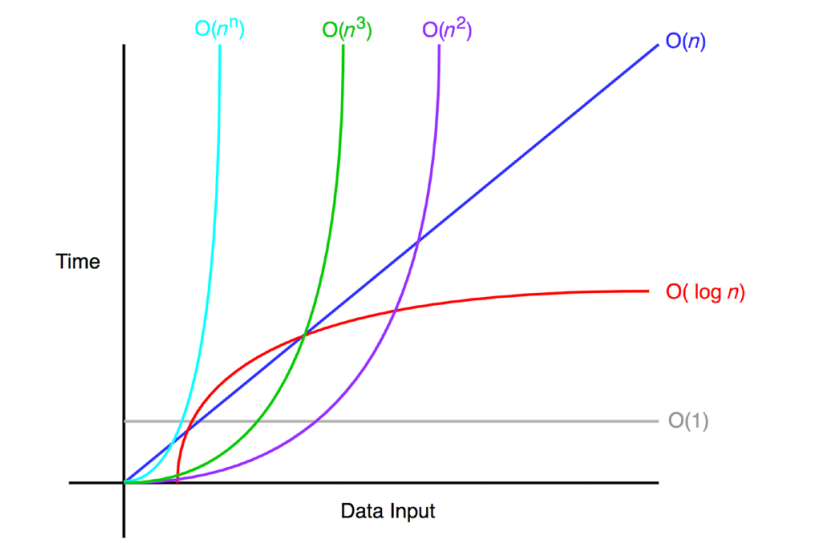# Algorithms – Time Complexity

Originally posted on my medium page: https://medium.com/@otarutundeSource: https://medium.com/better-programming/understanding-big-o-notation-c3245b8112dc

Time complexity is probably the most used term in the world of algorithms and data-structures. This article will introduce you gently into understanding time complexity also known as the Big Oh notation

Understanding the Basics

Every code you write in a programming language is always transformed to binary instructions on the computer. A CPU (Central Processing Unit) in a computer executes these instructions.

The CPU includes:

• ALU (Arithmetic Logic Unit) : performs arithmetic and logic operations
• Process Registers : that supplies operands to the ALU and store the results from the ALU operations.
• Control Unit : coordinates all actions between components in the CPU.

To make things simpler, let us abstract the operations a computer can perform to be:

• Assignment operation
• Arithmetic operation
• Comparator Operation

Using this code snippet as an example below:

```for (i = 0; i =< n; i++) {
m += 5;
}```

Using the operations listed above, the following are the steps the computer will perform below:

• Assign 0 to i
• Compare i to n
• Continue steps below if i =< n,
• Add 5 to m
• Increment i by 1

— it is clearly seen that this process continues until i > n

Now from the above, if n = 0, the number of instructions executed by the computer will be 5.

if n = 1, the computer will execute 5 * 2 = 10 instructions.

if n = 2, the computer would execute 5 * 3 = 15 instructions.

We can create a function for the number of instructions to be executed for n in the program above to be: f(n) = 5(n+1)

Big Oh Notation

The Big Oh tells how a function behaves as the size of the input approaches infinity (as the input grows very large).

In our case above, the input is n , if we assume n to be (100,000,000), the computer will perform 5(100,000,000 + 1) operations. We can afford to remove smaller terms from the equation as n grows very large because they become insignificant. We remove the constants 5 and 1 to make f(n) = n.

The function f(n) = n, is a linear function and thus can confidently say the time complexity for the operation above is in linear time O(n).

The function f(n) = n, is a linear function and can clearly see that this algorithm executes in linear time as n grows, this is also called O(n) in the big Oh notation. There are other Big Oh Notations derived from the same process above:

f(n) = n² — quadratic time O(n²)

f(n) = log n— logarithmic time O(log n)

and so on….

More Resources